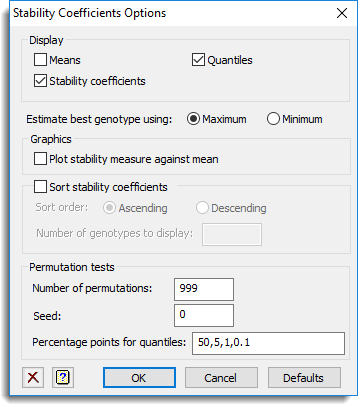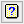1. Home
2. Stability Coefficients Options

# Stability Coefficients Options

Use this to select options and output to be used by calculating stability coefficients.## Display

Specifies which items of output are to be displayed in the Output window.

 Means The overall means of the genotypes. Stability coefficients The stability coefficients for each stability measure. Quantiles The quantiles for the permutation tests.

## Estimate best genotype using

Specifies whether the best genotype is defined to be the one with the Maximum mean yield or the one with the Minimum mean yield.

## Graphics

This specifies whether plots are to be produced by the analysis.

 Plot stability measure against mean Use this to plot the stabilities against the mean responses. This provides a way of simultaneously assessing the general effectiveness and stability of the genotypes.

## Sort stability coefficients

When selected, the stability coefficients for each selected method will be sorted, based on their stability, and displayed in the output. The following options can be used to control how the stability coefficients are displayed:

 Sort order This specifies the direction for the sort. Select Ascending to sort in ascending order of the most stable genotypes and Descending to sort in descending order. Number of genotypes to display This can be used to control the number of genotypes that are sorted and included in the display within the output. If this field is left blank, then all the genotypes will be included in the output.

## Permutation tests

In the permutation tests the original data are randomly permuted within each environment and the stability coefficients are calculated and stored. The analysis constructs a variate Group to indicate genotypes that occurred in exactly the same sets of environments: you cannot make comparisons between genotypes that occurred at different sites as these will have been competing with different genotypes across their environments. The permuted and original coefficients are combined within each group, and quantiles are calculated over the combined set of values. A coefficient can then be taken as significant at a particular level if its coefficient is greater than the corresponding quantile.

 Number of permutations Specifies how many permutations are done for the test. Seed Specifies the seed used to generate the random numbers used to generate the permutations. The default value of zero initializes the seed at random if this is the first time that the Genstat randomization routines have been used in the current job; otherwise it continues the existing sequence of random numbers. Percentage points for quantiles Provides a space to specify a single value or list of values separated by commas to define which quantiles are calculated.

## Action buttons

 OK Save the option settings and close the dialog. Cancel Close the dialog without making any changes. Defaults Resets options to their default settings.

## Action IconsClear Clear all fields and list boxes.Help Open the Help topic for this dialog.